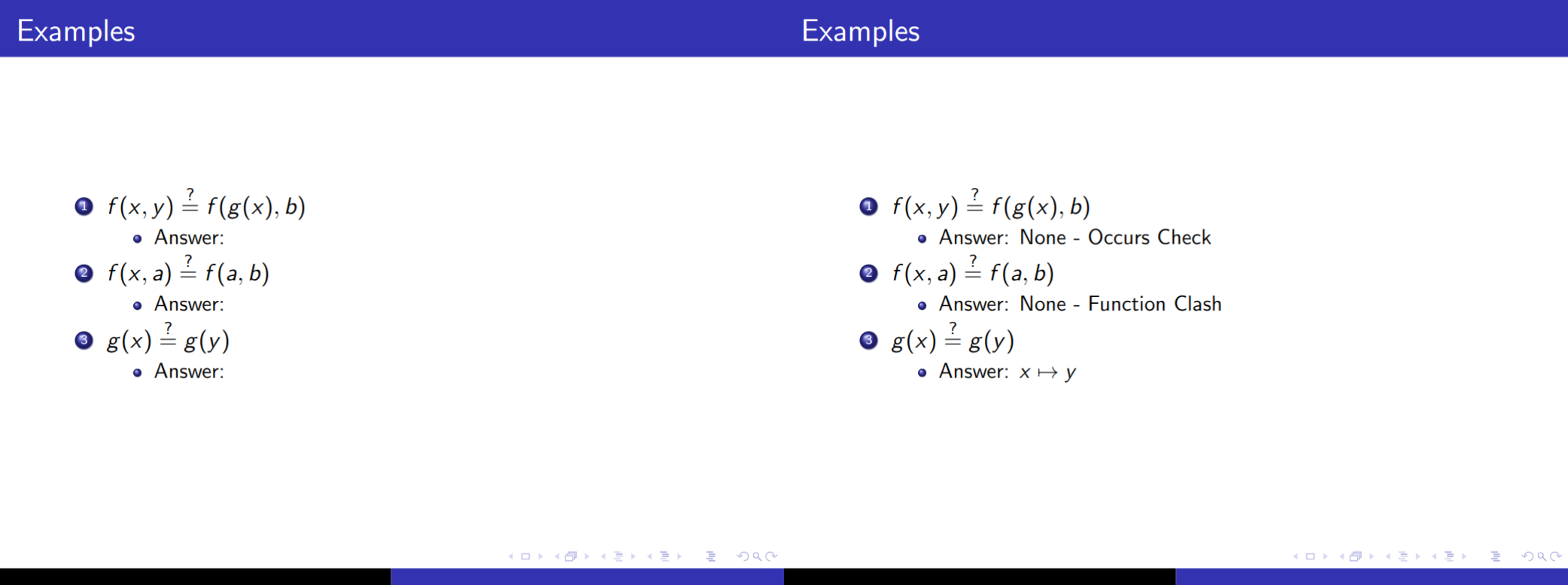# ~/Blog

## Brandon RozekPhD Student @ RPI studying Automated Reasoning in AI and Linux Enthusiast.

# Hiding Answers in Lecture Slides using Latex Beamer

Published on

Asking questions is a great way to try to solicitate engagement from students. However, it can feel at odds with trying to provide a fully comprehensive slide deck at times. Similar to having notes hidden for yourself, we should also have a way to hide answers for the students of the present but not the students of the future.

LaTex beamer has the \invisible command which holds the space for the text but doesn’t display it. We can create a new command with an if statement stating whether or not we want to use \invisible.

For this, I called the variable showanswer, but you can call it whatever you like. Put the following code in the preamble of your beamer document.

\newif\ifshowanswer


To write if not in LaTex, we need to use \unless\if.

Now let’s see this in action. Here is a slide I gave in a recent lecture about Unification:

\begin{frame}{Examples}
\begin{enumerate}
\item $f(x, y) \eq f(g(x), b)$
\begin{itemize}
\item Answer: \HideAns{None - Occurs Check}
\end{itemize}

\item $f(x, a) \eq f(a, b)$
\begin{itemize}
\item Answer: \HideAns{None - Function Clash}
\end{itemize}

\item $g(x) \eq g(y)$
\begin{itemize}
\item Answer: \HideAns{$x \mapsto y$}
\end{itemize}
\end{enumerate}
\end{frame}


The left shows the hidden version, and the right shows the version I can distribute afterwards.Minimal Working Example:

\documentclass{beamer}
\usetheme{Copenhagen}
\usepackage[utf8]{inputenc}

\newcommand{\eq}{\stackrel{?}{=}}

\begin{document}
\begin{frame}{Examples}
\begin{enumerate}
\item $f(x, y) \eq f(g(x), b)$
\begin{itemize}
\item Answer: \HideAns{None - Occurs Check}
\end{itemize}
\item $f(x, a) \eq f(a, b)$
\begin{itemize}
\item Answer: \HideAns{None - Function Clash}
\end{itemize}
\item $g(x) \eq g(y)$
\begin{itemize}
\item Answer: \HideAns{$x \mapsto y$}
\end{itemize}
\end{enumerate}
\end{frame}
\end{document}